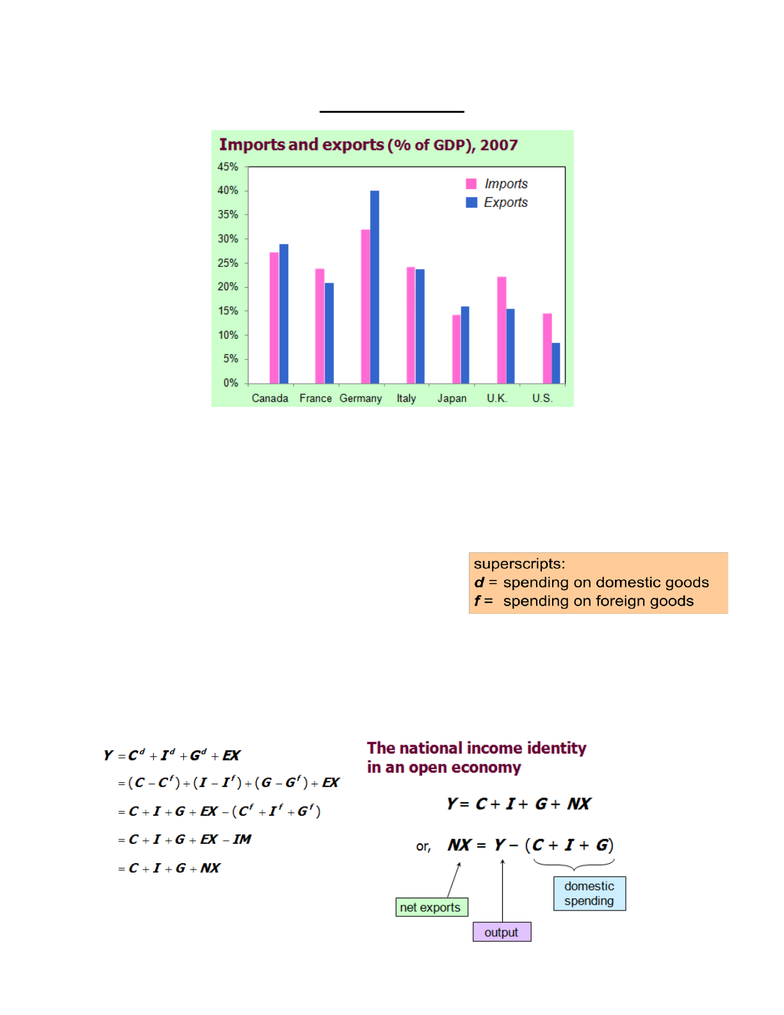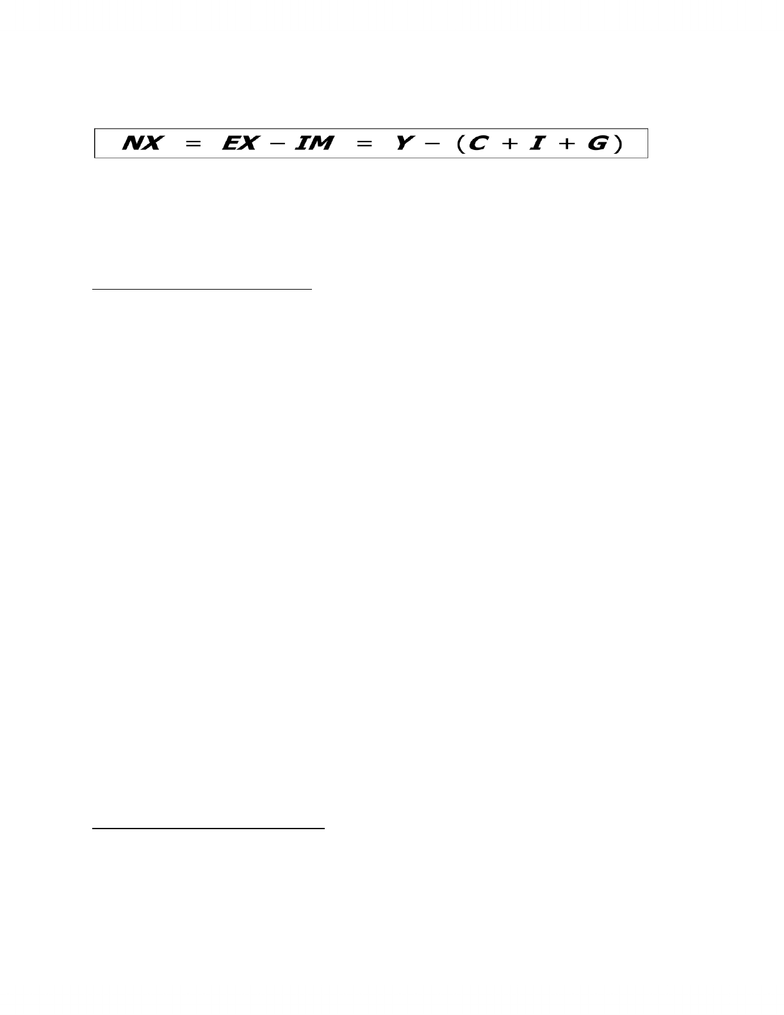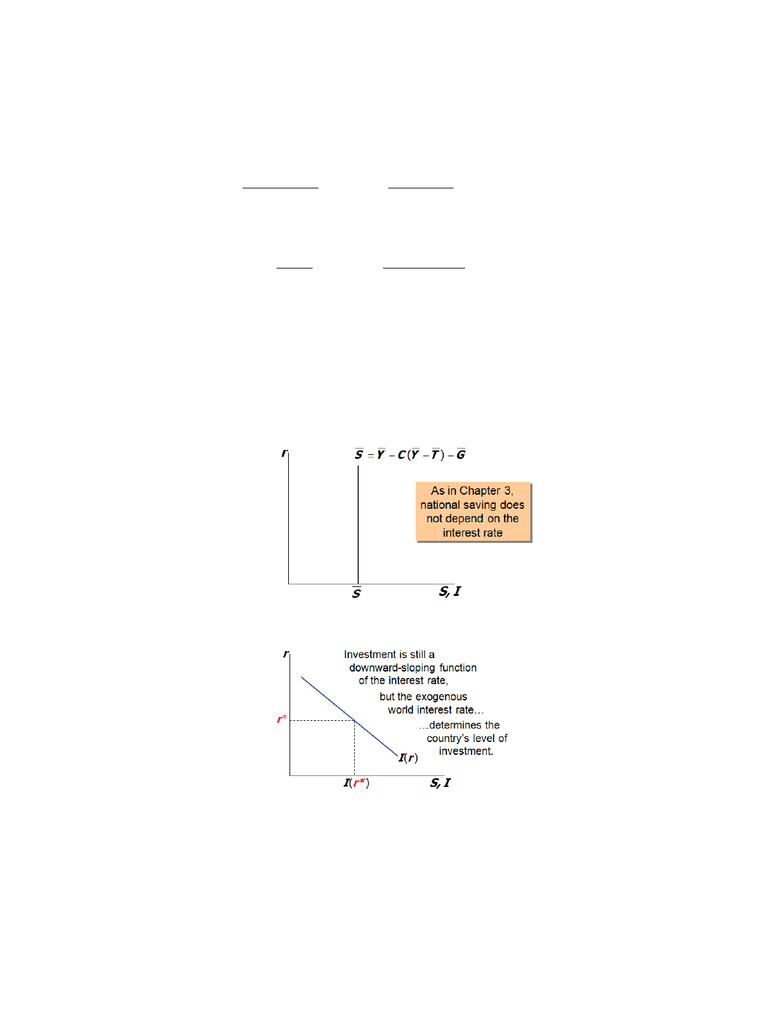Study Guides (400,000)
CA (160,000)
ECON (4)
Final

# ECON202 Study Guide - Final Guide: Import Quota, Real Interest Rate, Vestment

Department
Economics
Course Code
ECON202
Professor
Maryann Vaughan
Study Guide
Final

This preview shows pages 1-3. to view the full 14 pages of the document.The Open Economy
Canada has a very open economy
In an Open Economy
spending need not equal output
saving need not equal investment
Preliminaries
EX = exports = foreign spending on domestic goods
IM = imports = C f + I f + G f = spending on foreign goods
NX = net exports (a.k.a. the “trade balance”) = EX IM
GDP = Expenditure on Domestically Produced Goods and Services
df
C C C

df
I I I

df
G G G


Only pages 1-3 are available for preview. Some parts have been intentionally blurred.trade surplus: output > spending and exports > imports
Size of the trade surplus = NX
trade deficit: spending > output and imports > exports
Size of the trade deficit = NX
From the National Accounting Identity
Y = C + I + G+ NX
Subtract C and G from both sides:
Y C G = I + NX
Recall: S = Y C G
Therefore: S = I + NX
Solve for NX: NX = S I
Trade Balance = Excess of savings over investment
International Capital Flows
Net capital outflow
o = S I
o = net outflow of “loanable funds”
o = net purchases of foreign assets
the country’s purchases of foreign assets
minus foreign purchases of domestic assets
When S > I, country is a net lender
When S < I, country is a net borrower
NX = S I
S I is the difference between domestic savings and domestic investment
S I is called the Net Capital Outflow (NCO)
NCO = amount of foreign lending amount of foreign borrowing
Since: NX = S I and
o NCO = S I, then
o NX = NCO
The Link between NX = Y (C + I + G )
NX = Y (C + I + G) implies NX = (Y C G) I
o = S I
trade balance = net capital outflow

Only pages 1-3 are available for preview. Some parts have been intentionally blurred.Thus, a country with a trade deficit (NX < 0) is a net borrower (S < I ).
If (S I) > 0
o That implies: S > I
o Therefore, Exports > Imports
o There is a trade surplus and we are net lenders.
If (S - I) < 0
o That implies: S < I
o Therefore, Exports < Imports
o There is a trade deficit and we are net borrowers.
a. Domestic and foreign bonds and perfect substitutes (same risk, maturity, etc)
b. Perfect capital mobility: no restriction on international trade in assets
c. Economy is small: cannot affect the world interest rate, denoted r*
A and B imply r = r*, C implies r* is exogenous.
National Saving: The Supply of Loanable Funds
Investment: the Demand for Loanable Funds